• 方程求解程序清单 a=-1,b=2,c=-1; w=1; m=2; n=1; h = 0.02; t=0:h:30; s1=dsolve('a*D2y+b*Dy+c*y=sin(w*t)','y(0)=m,Dy(0)=n','t'); s1_n = eval(s1); hold on plot(t,s1_n,'ko'); EulerOED(a,b,c,w,m,n,h); hold ...
方程求解程序清单 a=-1,b=2,c=-1; w=1; m=2; n=1; h = 0.02; t=0:h:30; s1=dsolve('a*D2y+b*Dy+c*y=sin(w*t)','y(0)=m,Dy(0)=n','t'); s1_n = eval(s1); hold on plot(t,s1_n,'ko'); EulerOED(a,b,c,w,m,n,h); hold off  function EulerOED(a,b,c,w,x0,x1,h) A = [x0;x1]; t=0:h:30; for i = 1:1:length(t)-1 A(:,i+1) = [1,h;(-(c/a)*h),(1-(b/a)*h)]*A(:,i) + [0;(h/a)]*sin(w*t(i)); end plot(t,A(1,:),'r*'); 对于二阶全微分方程a*y''(t)+b*y'(t)+c=sin(wt) ，不同的a,b,c,w取值和初始条件会求出不同的解，通解又是由齐次解和特解组成。其中，齐次解由特征方程决定，而特解的决定因素则比较复杂。 讨论思路     (1)通解随初始条件变化情况     (2)通解随a,b,c变化情况              b^2-4ac>0(两个不同的实根)              b^2-4ac=0(两个相同的重根)              b^2-4ac<0(两个不同的复数根)  1).b>0  2).b=0  3).b<0      (3)通解随w变化情况                    b^2-4ac=0情况    b^2-4ac<0情况 (3)通解随w变化的规律 W属于(0,1)时，随w的增大在齐次解的旁边波动 w属于(1，+)，随w的增大逐渐趋近于齐次解。 Matlab解二阶常微分方程 方程：a*y''(t)+b*y'(t)+c=sin(wt)  求解：1.解析解                2.数值解(欧拉方法)  目的：1.比较两种求解方式的拟合情况             2.通解随w变化的规律 (1)通解随初始条件变化情况       Ex1:  a=2,b=3,c=1,y(0)=0;y'(0)=0,w=1   Ex2:  a=2,b=3,c=1,y(0)=2;y'(0)=0,w=1   Ex3:  a=2,b=3,c=1,y(0)=2;y'(0)=4,w=1  (2)通解随a,b,c变化情况       Ex1:  a=2,b=3,c=1,y(0)=0;y'(0)=0,w=1    Ex4:  a=-2,b=3,c=1,y(0)=0;y'(0)=0,w=1   Ex5:  a=2,b=-3,c=1,y(0)=0;y'(0)=0,w=1  Ex6:  a=2,b=3,c=-1,y(0)=2y'(0)=1,w=1 ?  EX: a=2 ,b=2*sqrt(2) ,c=1,y(0)=0;y'(0)=0,w=1   (3).b^2-4ac<0       EX:a=4,b=-1,c=2,y(0)=0;y'(0)=0,w=1  EX:a=4,b=1,c=2,y(0)=3,y'(0)=0,w=1  EX:a=4,b=0,c=1,y(0)=2;y'(0)=0,w=1
展开全文• 在解如图所示的方程时：!... 求解x在[0 1]范围内的数值解。 直接使用ode45函数时，未知C(0)值。...使用bvp4c函数时，又不知道如何输入控制一阶...请教如何在matlab中能够在已知一阶导数和终端数值的数值解的方法思路。开发语言
• MATLAB 求解微分方程x=dsolve('Dx=r*(1-x/xm)*x','x(0)=x0','t')x=xm/(1+exp(-r*t)*(xm-x0)/x0)用matlab求解这个微分方程：dx/dt=36.86+xx=dsolve('Dx=36.86+x')x=-1843/50+exp(t)*C1单摆微分方程求解：x''+(g/l)sin...
MATLAB 求解微分方程x=dsolve('Dx=r*(1-x/xm)*x','x(0)=x0','t')x=xm/(1+exp(-r*t)*(xm-x0)/x0)用matlab求解这个微分方程：dx/dt=36.86+xx=dsolve('Dx=36.86+x')x=-1843/50+exp(t)*C1单摆微分方程求解：x''+(g/l)sin(x)=0,用Matlab求解,1.这段程序基本没有什么错误,只是在最后调用ode45求解时候,格式有点错误,修改一下就能运行了：[t,x]=ode45(@Pendel_DGL,[0,4],[pi/2,0])2. 在编程时MATLAB 求解微分方程数值解结果：代码：clearallclcf=@(x,y)([y(2);   0.357*y(1)-0.1905*y(1)*y(2)]);[x,Y]=ode45(f,求解二阶微分方程∵齐次方程y''-6y'+9y=0的特征方程是r²-6r+9=0,则r=3(二重根)∴此齐次方程的通解是y=(C1x+C2)e^(3x)(C1,C2是积分常数)∵设原方程的解为y=(Ax用Matlab编程求解 二阶微分方程：4*d^2y(t)/dt^2+y(t)=dx(t)/d(t)-0.5x(t)MATLAB提供了dsolve命令可以用于对符号常微分方程进行求解.语法：dsolve(‘eq’,’con’,’v’)%求解微分方程dsolve(‘eq1,eq2…’,’con1,con2…’,’v1利用MATLAB求解微分方程初值问题朋友,要根据初值积分对dM/dt积分求得M,才能求解M(t)=0或t(M)=0.solver(积分函数,积分时间,初值,设置)也是这样的数学方法.[时间,解]=solver(积分函数,积分时间,初值)matlab求解微分方程并画图symstv=dsolve('Dv=(190.708-90.64*v^2)/47.27','v(0)=0','t');t=0:0.00001:0.002;v=eval(v);plot(t,v)使用这样MATLAB 求解微分方程的错误看了看,运行了一下确实出问题 原因是在用ode数值求解时,x并不是1:0.01:3均匀分散的 解决方法：1.在画解析解和欧拉解时横轴用x的转置；在画数值解时横轴用x,已运行成功2.matlab求解微分方程的问题我运行的>>symsaknNzz1>>z=dsolve('Dx=a*x*(N-x)','t')z1=dsolve('Dx=a*x*(n-x)','t')结果：z=N0N/(exp(-N*(C3+a*tmatlab ode45 求解二阶常微分方程functiontest()[t,y]=ode45(@func1,[0,1],[0;0;1;2;2;2]);figure(1);clf;plot(t,y);legend('x','y','z','dxmatlab里的ode45求解二阶微分方程问题!新的matlab版本好像不鼓励采用global了.你的全局变量有点多了,哈哈.简单例子:m=2;[t,y]=ode45(@(t,x)f1(t,x,m),[0,10],)functiondy=f1matlab怎么求解偏微分方程Matlab偏微分方程工具箱应用简介1．概述本文只给出该工具箱的函数列表,读者应先具备偏微分方程的基本知识,然后根据本文列出的函数查阅Matlab的帮助,便可掌握该工具箱的使用.2．偏微分方程算法函数matlab 求解一阶偏微分方程i是虚数单位?那个1/3γ(u*v)中*是什么符号用matlab求解二阶微分方程数值解,程序出现错误,求大神指点1、把G=1/3*((5*Pp-2*P1)/(P1-2*Pp)-P1*c1^2/Pp*cp^2);改成G=1/3*((5*Pp-2*P1)/(P1+2*Pp)-P1*c1^2/(Pp*cp^2));表matlab 矩阵微分方程求解最常用的就是广义特征向量基础矩阵解方法.你要一个思路,我给一个2维情况的例子,其中特解x(t0)=x0的理解和如何使用都有,你看看是否够用.. Matlab下二维的例子：再问：嗯，这个不错，用MATLAB求解9阶微分方程用什么函数可以不管多少阶都可以用ode45,注意把高阶化成一阶即可,比如一个9阶微分方程可化为9个一阶方程,具体方法可网上找,很简单的matlab数值解法求解二阶微分方程 ODE45函数因为你x=0时2/x是无穷大呀,然后y'又是0,然后(2/x)y'就是nan了,所以后面算的全是nan了.用matlab方程求解微分方程y=dsolve('2000*Dy-(0.08-y*0.08)','y(0)=0','t')y=1-exp(-1/25000*t)即：C(t)=1-exp(-1/25000*t)
展开全文• x(t) r(t) 两个关于t的函数 下面两个方程对t求二阶导，...我看MATLAB微分方程一般都是第一个式子的形式，第二个式子的左边有两个函数的话，能解吗？能的话，该怎么写程序呢？ x ''=2x+r ((x^2+r^2)^0.5 )''=2r+x开发语言
• M=[2,0;0 1 ]; %质量矩阵K=[6 -2;-2 4]; %刚度矩阵a=0;b=0;C=a*K+b*M;dt=0.28;t=0:dt:2.8;ft0=zeros(length(K),length(t));for i=1:length(t)ft0(1,i)=10;%在节点4的竖直方向加大小为200N的阶跃力e...
M=[2,0;0 1 ];                      %质量矩阵K=[6 -2;-2 4];                   %刚度矩阵a=0;b=0;C=a*K+b*M;dt=0.28;t=0:dt:2.8;ft0=zeros(length(K),length(t));for i=1:length(t)ft0(1,i)=10;  %在节点4的竖直方向加大小为200N的阶跃力enddsp=zeros(length(K),length(t));                                         % 位移vel=zeros(length(K),length(t));                                             % 速度acc=zeros(length(K),length(t));                                          % 加速度%--------------------------------------------------------------------------%  (2) Newmarkalpha=0.3; beta=0.6;                                        % 稳定条件acc(:,1)=inv(M)*(ft0(:,1)-K*dsp(:,1)-C*vel(:,1));   % 计算初始加速度 (t=0)ekk=K+M/(alpha*dt^2)+C*beta/(alpha*dt);  % 计算有效刚度矩阵for it=2:length(t)-1                                              % 时间步循环cfm=dsp(:,it)/(alpha*dt^2)+vel(:,it)/(alpha*dt)+acc(:,it)*(0.5/alpha-1);cfc=dsp(:,it)*beta/(alpha*dt)+vel(:,it)*(beta/alpha-1)...+acc(:,it)*(0.5*beta/alpha-1)*dt;efd=ft0(:,it)+M*cfm+C*cfc;                  %  计算有效力矢量dsp(:,it+1)=inv(ekk)*efd;                        %  t+dt时刻的位移acc(:,it+1)=(dsp(:,it+1)-dsp(:,it))/(alpha*dt^2)-vel(:,it)/(alpha*dt)...-acc(:,it)*(0.5/alpha-1);              %  t+dt时刻的加速度vel(:,it+1)=vel(:,it)+acc(:,it)*(1-beta)*dt+acc(:,it+1)*beta*dt; %  t+dt时刻的速度endplot(t, dsp(2,:),'-b*')hold onxlabel('Time(seconds)')ylabel(' Vertical displ. (m)')%  (2) Wilson%--------------------------------------------------------------------------theta=1.4;                                          % 稳定条件参数acc(:,1)=inv(M)*(ft0(:,1)-K*dsp(:,1)-C*vel(:,1)); % 计算初始加速度 (t=0)ekk=K+M*(6/(theta*dt)^2)+C*(3/(theta*dt));  % 计算有效刚度矩阵for it=2:length(t)-1cfm=dsp(:,it)*(6/(theta*dt)^2)+vel(:,it)*(6/(theta*dt))+2*acc(:,it);cfc=dsp(:,it)*(3/(theta*dt))+2*vel(:,it)+acc(:,it)*(theta*dt/2);efd=ft0(:,it)+theta*(ft0(:,it+1)-ft0(:,it))+M*cfm+C*cfc; %计算有效力矢量dtheta=inv(ekk)*efd;                         %  t+ dt时刻的位移acc(:,it+1)=(dtheta-dsp(:,it))*(6/(theta^3*dt^2))...-vel(:,it)*(6/(theta^2*dt))+acc(:,it)*(1-3/theta);  %  t+dt时刻的加速度vel(:,it+1)=vel(:,it)+acc(:,it+1)*dt/2+acc(:,it)*dt/2; %  t+dt时刻的速度dsp(:,it+1)=dsp(:,it)+vel(:,it)*dt...+(acc(:,it+1)+2*acc(:,it))*(dt^2/6);         %  t+dt时刻的位移endplot(t, dsp(2,:),'-g*')hold onxlabel('Time(seconds)')ylabel(' Vertical displ. (m)')%--------------------------------------------------------------------------%  (2) 振型叠加法%施加不平衡激振力t=t';[V,D]=eig(K,M);                       % 计算特征值和特征向量[lambda,ki]=sort(diag(D));                     % 给特征值和特征向量排序omega=sqrt(lambda);                                       % 角频率w.omega1=sqrt(lambda)/(2*pi);                           % 固有频率 Hz.V1=V(:,ki);h=V1'*M*V1;Factor=diag(V1'*M*V1);                             %模态质量Vnorm=V1*inv(sqrt(diag(Factor)));                  % 正则化振型向量VnormK=diag(Vnorm'*K*Vnorm);                       %模态刚度omega0=diag(sqrt(Vnorm'*K*Vnorm)) ;                % 正则化模态刚度VnormM=diag(Vnorm'*M*Vnorm);                       %正则化模态质量Fnorm=Vnorm'*ft0 ;                                     % 模态力矢量Modamp=Vnorm'*(a*M+b*K)*Vnorm;                     % 模态阻尼矩阵zeta=diag((1/2)*Modamp*inv(diag(omega0)));           % 阻尼比%--------------------------------------------------------------------------%  %  (4) 模态坐标的响应%--------------------------------------------------------------------------q0=zeros(length(K),1);dq0=zeros(length(K),1);                         % 初始化位移和速度eta0=Vnorm'*M*q0; deta0=Vnorm'*M*dq0;     %2.2-53% 初始条件模态坐标的位移和速度eta=zeros(length(t),length(K));                %  %初始化位移2.2-52for i=1:length(K)                                 % t(i)时刻的响应phase0=omega0(i)*t;                                %w*t omega0为无阻尼固有频率omegad=omega(i)*sqrt(1-zeta(i)^2);phase=omegad*t;                            %wd*t其中(omegad为有阻尼固有频率)Exx=exp(-zeta(i)*omega(i)*t);                %中间变量C1=eta0(i);                                        %初始位移C2=(deta0(i)+eta0(i)*zeta(i)*omega0(i))/omegad;          %中间变量D1=zeta(i)*omega(i)/omegad;II=ones(length(t),1);XX=Fnorm(i)/(omegad^2+zeta(i)^2*omega(i)^2);eta(:,i)=C1*Exx.*cos(phase)+C2*Exx.*sin(phase)+ XX*(II-Exx.*cos(phase)-D1*Exx.*sin(phase));%有阻尼系统%  eta(:,i)=Fnorm(i)/((omega(i))^2)*(1-cos(omega(i)*t)); %无阻尼系统end%--------------------------------------------------------------------------%   (6) 将模态坐标转化到物理坐标系%--------------------------------------------------------------------------eta=eta';            %模态坐标y=Vnorm*eta;           %物理坐标plot(t, y(2,:),'-r*')xlabel('Time(seconds)')ylabel(' Vertical displ. (m)')
展开全文• 我们常常用微分方程来描述现实世界中的一些物理现象。由于微分方程的复杂性，只有在很简单的情况下才能得到微分方程的解析解。由于计算机的发展，采用数值方法求解微分方程的数值近似解得到广泛应用。微分方程的数值...
我们常常用微分方程来描述现实世界中的一些物理现象。由于微分方程的复杂性，只有在很简单的情况下才能得到微分方程的解析解。由于计算机的发展，采用数值方法求解微分方程的数值近似解得到广泛应用。微分方程的数值解法主要包括两大类：有限差分法和有限单元法。这里主要介绍有限单元法。

However，对于一个只学过微积分和矩阵论的工科生来说，要了解有限元法的数学原理还是有些困难，所以这里重点是介绍有限元法的思想和具体计算方法，深层的数学原理并不涉及，主要是本人也不懂~~~

1.微分方程基本概念

微分方程主要分为常微分方程和偏微分方程。
常微分方程：未知函数是单一自变量的函数，按照方程中导数的阶数，分为一阶、二阶、n阶常微分方程。
偏微分方程：未知函数是多个自变量的函数，至少是两个自变量，同样可以分为一阶、二阶、n阶偏微分微分方程。

二阶偏微分方程研究较多，又可以分为椭圆型方程、双曲型方程、抛物型方程等。这里不展开，具体可以查看偏微分方程数值解法相关书籍。

2.二阶常微分方程的有限元法求解举例

由于下面涉及大量公式，所以采用截图的方式进行介绍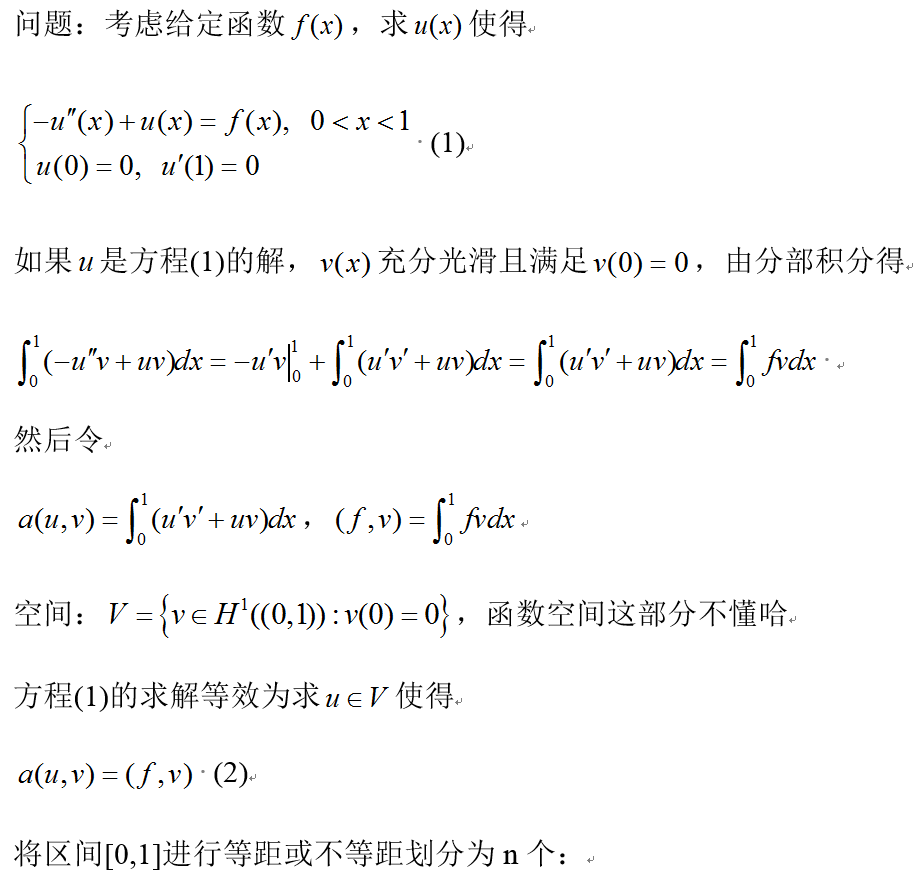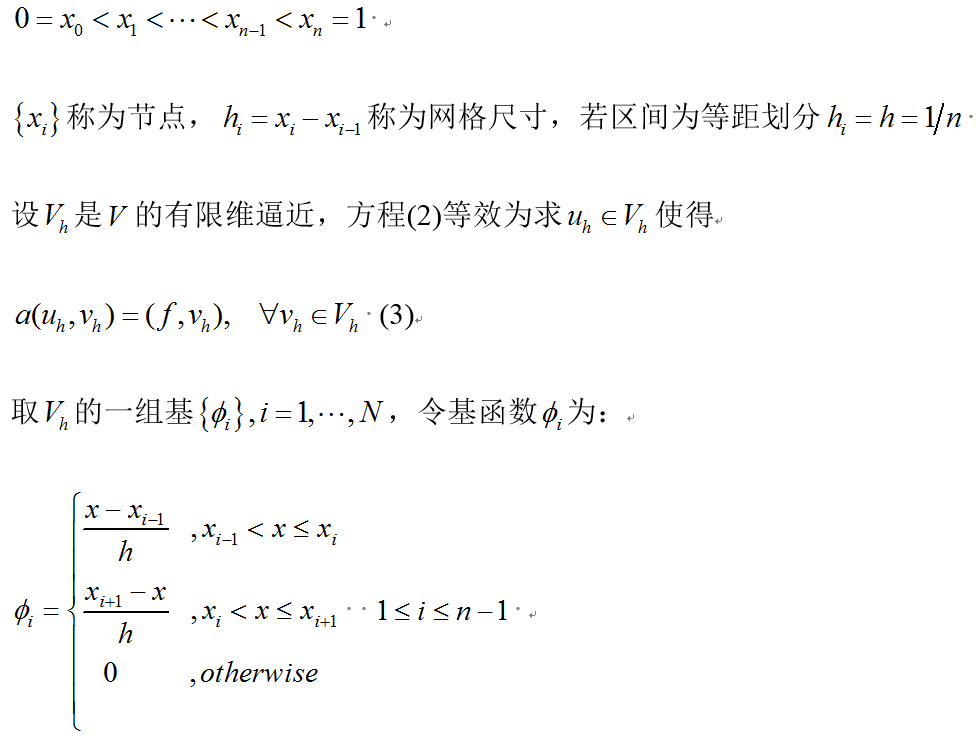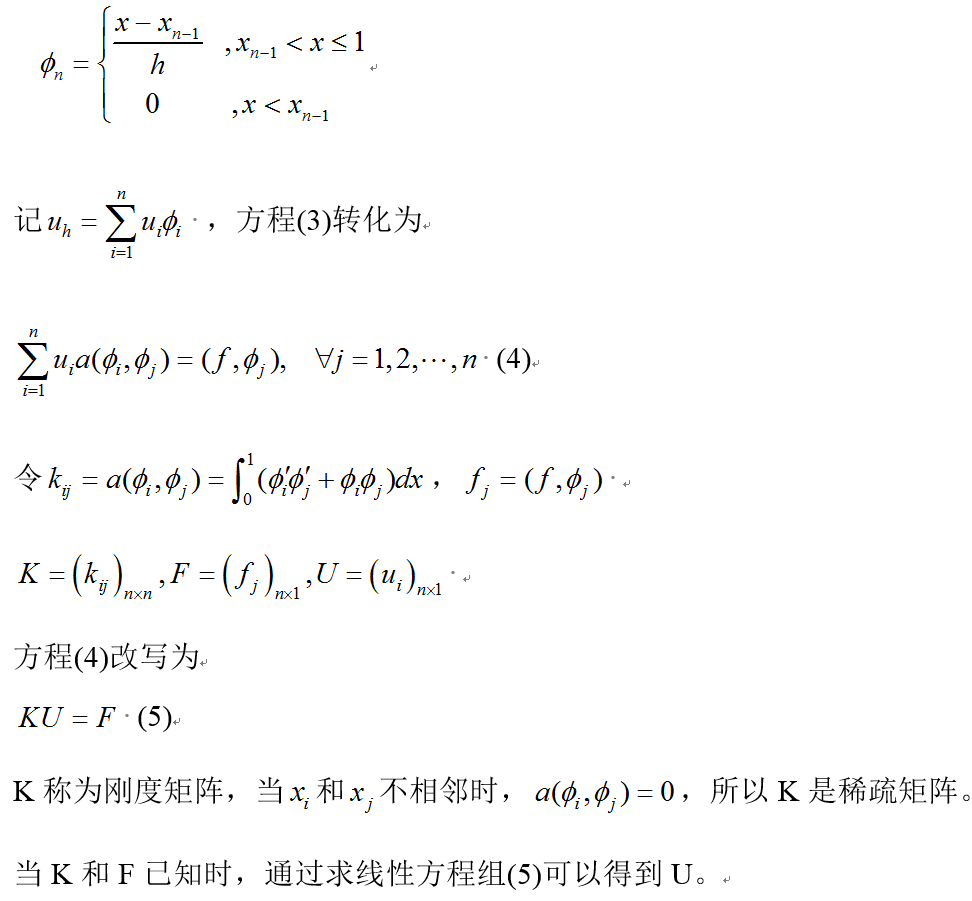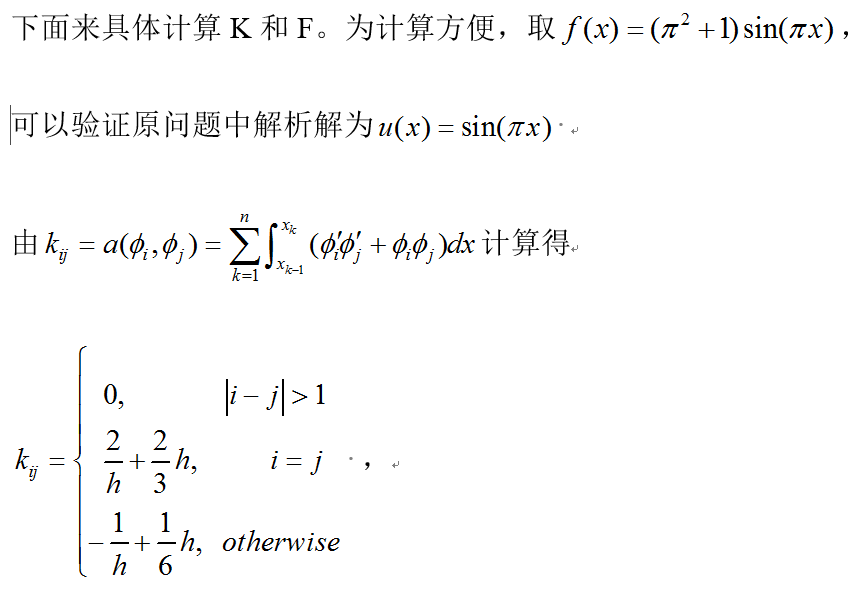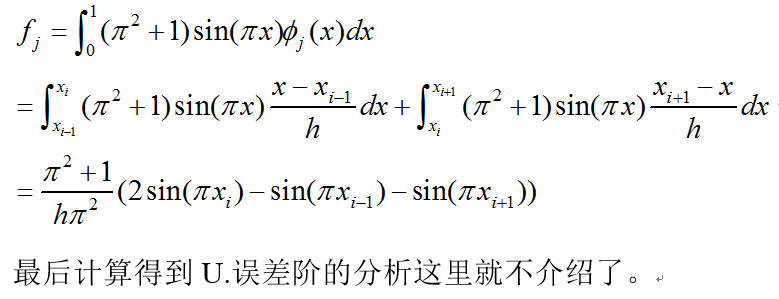3.具体Matlab实现如下：

%% main program
%一次有限元求一维常微分方程，基函数为分片线性函数
n = 10;
err = zeros(8, 1);

% Linear interpolation
index = zeros(8, 1);
for i=1:8
index(i) = 2^(i-1) * n; %10,20,40,80,160,320,640,1280
end
for i = 1:8
N = index(i); %网格数量
h = 1 / N; %网格大小
x = 0:h:1; %节点向量
xx = 0:1/1000:1;
exact_solu = sin(pi.*xx); %精确解
K = zeros(N-1); %初始化系数矩阵（刚度矩阵）
K = K + diag(ones(N-1,1).*(2/h+2/3*h), 0) + diag((h/6-1/h).*ones(N-2,1), 1)...
+ diag((h/6-1/h).*ones(N-2,1), -1);
F = (1+pi^2)/(h*pi^2) * (2.*sin(pi.*x(2:N))-sin(pi.*x(1:N-1))-sin(pi.*x(3:N+1)))';
K = sparse(K);
coeff = K\F; %Ax=b,matlab求法x=A\b
solu = [0; coeff; 0]; %u(x)节点解

%     figure()
subplot(3,3,i)
plot(x', solu, 'o--',xx', exact_solu, 'g--');
legend('numerical solution', 'exact solution')
xlabel('x')
ylabel('U');
xlim([-0.05,1.05])
ylim([-0.05,1.05]);
title(['numerical solution and exact solution N=',num2str(index(i))]);

% calculate the error
du = solu(2:N+1) - solu(1:N);
y1 = sin(2.*pi.*x);
dy1 = y1(2:N+1) - y1(1:N);
y2 = sin(pi.*x);
dy2 = y2(2:N+1) - y2(1:N);
error = pi^2*h/2*ones(1,N) + du'.^2/h + pi/4*dy1 - 2/h*dy2.*du';
err(i) = sqrt(sum(error));
end
% figure()
subplot(3,3,9)
plot(log2(err),'o--');
title('误差阶')
xlabel('N')
ylabel('$log_{2}Error$', 'Interpreter','LaTex')
xlim([0.8,8.2])

axis on;
set(gca,'xtick',1:1:8);
set(gca,'xticklabel',{5,10,20,40,80,160,320,640});

[order,~] = polyfit(1:1:(length(err)), log2(err)', 1);
disp(order)



最后计算结果如下图所示：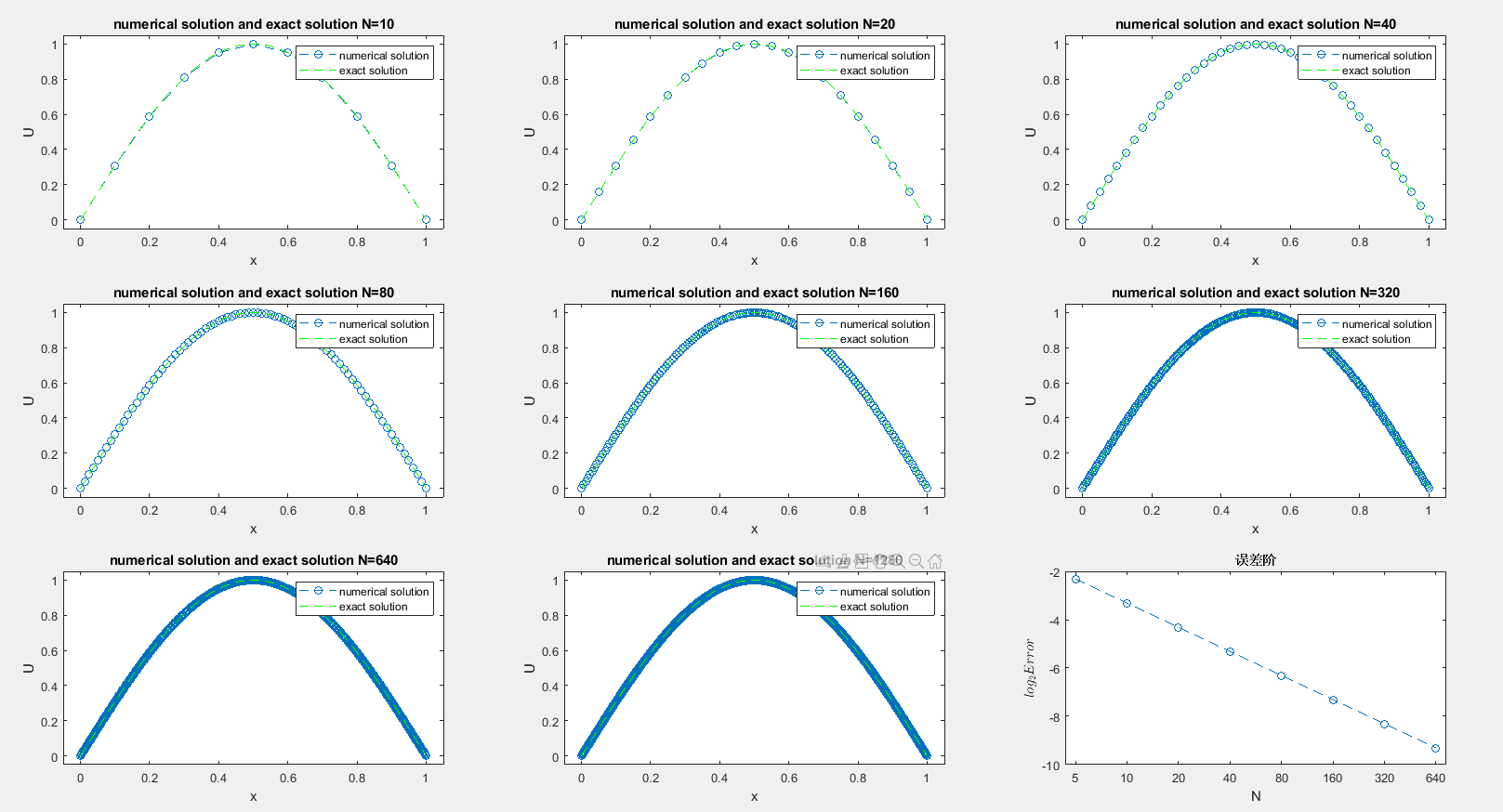参考文献：

代码 https://blog.csdn.net/waitingwinter/article/details/106164350

展开全文计算数学
• <p style="text-align:center"><img alt="" src="https://img-ask.csdnimg.cn/upload/1623239109110.jpg" /></p>  </p>有问必答
• 本文主要介绍matlab中求解常微分方程（组）的dsolve和ode系列函数，并通过例子加深读者的理解。 一、符号介绍  D: 微分符号；D2表示二阶微分，D3表示三阶微分，以此类推。 二、函数功能介绍及例程 1、dsolve ...
• 大意就是一个力学模型，现在需要解一个二阶微分方程但是很复杂，大概是有(D2y=f(y)的形式，f是一个由一些向量点乘、叉乘blabla得出来的以y为自变量的函数，但主要是三角函数与多项式、分式函数迭代，或者说，这个...
• 边值问题Matlab可用BVP4C命令，但感觉比较麻烦，下面用1stOpt求解，很简单快捷：CODE:Constant Pey=9.73, Nox=8.05, uxuy=3, bd=1, cx1e=2.3,b1=309.7,b2=2832.5, a1=27.8,a2=2.15,a3=-0.84,a4=0.935;Variable t=[0:...
• 本文主要介绍matlab中求解常微分方程（组）的dsolve和ode系列函数，并通过例子加深读者的理解。 一、符号介绍    D: 微分符号；D2表示二阶微分，D3表示三阶微分，以此类推。 二、函数功能介绍及例程 1、...
• 首先用高数知识求解非齐次常系数微分方程 再利用信号与系统中冲激响应求解验证 利用MATLAB求解验证 y=dsolve('D2y+3*Dy+2*y=exp(-t)','y(0)=1','Dy(0)=2','t') 得出结果： y = (t - 2 exp(-t) + 3) exp(-t) ...python
• 1）在表达微分方程时，用字母D表示微分，D2、D3分别表示二阶、三阶微分，后面跟的是求解的因变量； 2）自变量可以指定，不写时默认为t； 3）用单引号； 4）在单引号中的公式算数符号不能省略：比如‘y-Dy=2*x...
• ## MATLAB求解微分方程

千次阅读 多人点赞 2020-04-21 02:52:30
初始条件省缺时，是求微分方程的通解。 Dy代表y的导数，D2y代表y的二阶导数， D3y代表y的三阶导数…… 例1：求解微分方程： 解： y=dsolve('D2y-2*Dy+y-x^2=0','x') 例2： 解： y=dsolve('D2y+4*Dy+29*y','...
• ## Matlab学习——求解微分方程(组)

万次阅读 多人点赞 2018-05-29 17:18:58
函数 dsolve 用来解决常微分方程（组）的求解问题，调用格式为 X=dsolve(‘eqn1’,’eqn2’,…) 如果没有初始条件，则出通解，如果有初始条件，则出特解 系统缺省的自变量为 t。 2.函数d...
• 关于时间的微分方程,手工求解是相当复杂繁琐的,对于二阶动态电路,特别是一般二阶动态电路的求解就更为复杂.然而利用MATLAB来求解二阶动态电路问题,就可以变得简单,便捷.本文以具体实例讲述了MATLAB二阶动态电路...
• 文章目录前言一、欧拉法1.一阶微分方程2.二阶微分方程相空间总结：未完待续！ 前言 提示：这里可以添加本文要记录的...下面分别介绍欧拉法求解一阶微分方程，二阶微分方程matlab程序实现。 1.一阶微分方程 {dy(t)d
• x,y,z为位移，一阶导为速度，二阶导为加速度，m,F,c和两个角度为常数，用MATLAB求解微分方程组，![程序e](e\1.png)
• 2.在Mizar系统下,www.yifanglunwen.com/post/34.html首次实现了二阶微分的Mizar表述,定义的形式简洁明了,并完成了相应的运算公式和定理的Mizar证明与验证。3.将二元函数偏微分的理论推广到三维欧氏空间中,定义了...
• 方程组是这样的 y''= -10.01476+0.20811*（0.0587* x'-0.9983* y'） x''= -0.20811* (0.0587*y'+0.9983 * x') 都是二阶导和一阶导，在t=0时x为0，y为10600，不想要x,y关于t的曲线。想直接要x,y之间的曲线。 作图，...
• 文章目录1 MATLAB之极限、积分、微分2 matlab中微分方程的求解2.1 一阶微分方程2.2 求解二阶线性微分方程 是指含有未知函数及其导数的关系式。解微分方程就是找出未知函数。微分方程是伴随着微积分学一起发展起来的...
• 二阶RLC电路: 根据节点电压法出戴维宁等效电路，列写微分方程求得Uc，UL和t之间的关系，并绘图。 交互界面介绍 一阶RC全相应 Multisim仿真： 我们先用仿真软件Multisim绘制出待测电路图以及Uc-t曲线：
• ## matlab-双摆仿真

千次阅读 2018-12-29 20:45:00
matlab-双摆仿真 在物理学和数学中，在动力系统领域，双摆是一个摆锤，另一个摆锤连接在其...由于双摆运动涉及到二阶微分方程组，在matlab中对双摆的仿真需要用到ode45其数值解。 （代码来自h...
• 在物理学和数学中，在动力系统领域，...由于双摆运动涉及到二阶微分方程组，在matlab中对双摆的仿真需要用到ode45其数值解。clear all;%控制r1 r2两个角位移 就可改变双摆初状态r1=0.8;r2=0.8;m1=1;m2=1;L1=1;L2=1...
• 微分方程组的数值解： 欧拉法 龙格·库塔（Runge Kutta）二阶 龙格·库塔（Runge Kutta）4级 带边界条件的微分方程的射击方法 偏微分方程： 有限差分法：前进，后退，中央方案 Dirichlet边界条件，Newmann边界条件，...
• 一维二阶微分方程微分积法matlab代码，三种不同类型的非均匀节点插值函数并且和ode解法进行了对比。# matlab求二阶微分方程matlab 订阅